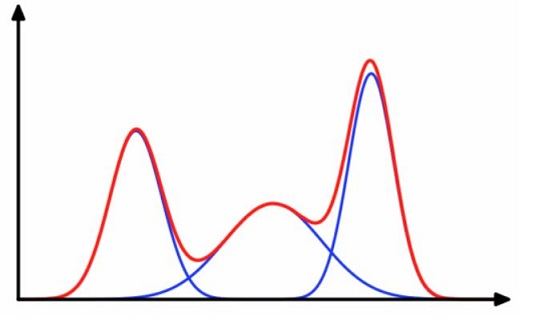# A Hardware Accelerator for Unsupervised Learning Based on a Gaussian Mixture ModelClustering is the task of dividing data points into a number of groups such that data points in the
same group are more similar to other data points in the same group than those in other groups.
Kmeans is an effective clustering algorithm based on clustering the data points using the
minimum distance of the mean of all the points in each cluster. For some datasets, Kmeans
does not provide the best results.
Gaussian Mixture Model is a universally used model for unsupervised learning or clustering.
GMM models are used for representing normally distributed sub-datasets within an overall dataset.
A gaussian or normal distribution s completely defined by its mean and standard deviation.
Using the probability density function, it is possible to calculate the probability (likelihood) of
a data point as being a part of the dataset represented by the function.
A Gaussian mixture model is a probabilistic model that assumes all the data points
belong to a mixture of a finite number of Gaussian distributions with initially unknown parameters
(in this case the means and the standard deviation).
The main difficulty when dealing with unlabeled data is that it it is not known which to gaussian
distribution the data points belong and the parameters of all the distributions, are also initially unknown.
Expectation-maximization is an excellent algorithm to get around this problem using an iterative process.
First one assumes how many clusters the data points represent. For each cluster a gaussian distribution
is formulated randomly (i.e. random mean and standard deviation).
In the next step the probability (or likelihood) of each data point belongs to each cluster is computed.
Based on these probabilities, the means and standard deviations are updated and the process is repeated
till convergence.
The goal of this project is to design and implement hardware to accelerate clusterin of data
based on the Gaussian Mixture Model. The students will learn the full flow of ASIC design
from specification to layout.